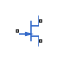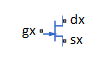# SPICE NJFET

SPICE-compatible N-Channel JFET

• Library:
• Simscape / Electrical / Additional Components / SPICE Semiconductors

•## Description

The SPICE NJFET block represents a SPICE-compatible N-channel junction field-effect transistor (NJFET). If the voltage applied to the gate port, gx, is less than the voltage applied to the source port, sx, the current between the source port and drain port, dx, is reduced.SPICE, or Simulation Program with Integrated Circuit Emphasis, is a simulation tool for electronic circuits. You can convert some SPICE subcircuits into equivalent Simscape™ Electrical™ models using the Environment Parameters block and SPICE-compatible blocks from the Additional Components library. For more information, see `subcircuit2ssc`.

### Equations

Variables for the SPICE NJFET block equations include:

• Variables that you define by specifying parameters for the SPICE NJFET block. The visibility of some of the parameters depends on the value that you set for other parameters. For more information, see Parameters.

• Geometry-adjusted variables, which depend on several values that you specify using parameters for the SPICE NJFET block. For more information, see Geometry-Adjusted Variables.

• Temperature, T, which is `300.15` `K` by default. You can use a different value by specifying parameters for the SPICE NJFET block or by specifying parameters for both the SPICE NJFET block and an Environment Parameters block. For more information, see Transistor Temperature.

• Minimal conductance, GMIN, which is `1e-12` `1/Ohm` by default. You can use a different value by specifying a parameter for an Environment Parameters block. For more information, see Minimal Conduction.

Several variables in the equations for the N-channel junction field-effect transistor model consider the geometry of the device that the block represents. These geometry-adjusted variables depend on variables that you define by specifying SPICE NJFET block parameters. The geometry-adjusted variables depend on these variables:

• AREA — Area of the device

• SCALE — Number of parallel connected devices

The table includes the geometry-adjusted variables and the defining equations.

VariableDescriptionEquation
`$BET{A}_{d}=BETA*AREA*SCALE$`
`$CG{D}_{d}=CGD*AREA*SCALE$`
`$CG{S}_{d}=CGS*AREA*SCALE$`
`$I{S}_{d}=IS*AREA*SCALE$`
`$R{S}_{d}=\frac{RS}{AREA*SCALE}$`
`$R{D}_{d}=\frac{RD}{AREA*SCALE}$`

Transistor Temperature

You can use these options to define transistor temperature, T:

• Fixed temperature — The block uses a temperature that is independent of the circuit temperature when the Model temperature dependence using parameter in the Temperature settings of the SPICE NJFET block is set to `Fixed temperature`. For this model, the block sets T equal to TFIXED.

• Device temperature — The block uses a temperature that depends on circuit temperature when the Model temperature dependence using parameter in the Temperature settings of the SPICE NJFET block is set to `Device temperature`. For this model, the block defines temperature as

`$T={T}_{C}+TOFFSET$`

Where:

• TC is the circuit temperature.

If there is not an Environment Parameters block in the circuit, TC is equal to 300.15 K.

If there is an Environment Parameters block in the circuit, TC is equal to the value that you specify for the Temperature parameter in the SPICE settings of the Environment Parameters block. The default value for the Temperature parameter is `300.15` `K`.

• TOFFSET is the offset local circuit temperature.

Minimal Conduction

Minimal conductance, GMIN, has a default value of `1e–12` `1/Ohm`. To specify a different value:

1. If there is not an Environment Parameters block in the transistor circuit, add one.

2. In the SPICE settings of the Environment Parameters block, specify the desired GMIN value for the GMIN parameter.

Gate-Source Current-Voltage Model

This table shows the equations that define the relationship between the gate-source current, Igs, and the gate-source voltage, Vgs. As applicable, the model parameters are first adjusted for temperature. For more information, see Temperature Dependence.

Applicable Range of Vgs ValuesCorresponding Igs Equation

${V}_{gs}>80*{V}_{t}$

${I}_{gs}=I{S}_{d}*\left(\left(\frac{{V}_{gs}}{{V}_{t}}-79\right){e}^{80}-1\right)+{V}_{gs}*G\mathrm{min}$

$80*{V}_{t}\ge {V}_{gs}$

${I}_{gs}=I{S}_{d}*\left({e}^{{V}_{gs}/{V}_{t}}-1\right)+{V}_{gs}*G\mathrm{min}$

Where:

• ISd is the geometry-adjusted saturation current.

• Vt is the thermal voltage, such that ${V}_{t}=ND*k*T/q$.

• ND is the emission coefficient.

• q is the elementary charge on an electron.

• k is the Boltzmann constant.

• T is the transistor temperature. For more information, see Transistor Temperature

• GMIN is the transistor minimum conductance. or more information, see Minimal Conduction

Gate-Drain Current-Voltage Model

This table shows the relationship between the gate-drain current, Igd, and the gate-drain voltage, Vgd. As applicable, model parameters are first adjusted for temperature.

Applicable Range of Vgd ValuesCorresponding Igd Equation

${V}_{gd}>80*{V}_{t}$

${I}_{gd}=I{S}_{d}*\left(\left(\frac{{V}_{gd}}{{V}_{t}}-79\right){e}^{80}-1\right)+{V}_{gd}*G\mathrm{min}$

$80*{V}_{t}\ge {V}_{gd}$

${I}_{gd}=I{S}_{d}*\left({e}^{{V}_{gd}/{V}_{t}}-1\right)+{V}_{gd}*G\mathrm{min}$

Drain-Source Current-Voltage Model

This table shows the relationship between the drain-source current, Ids, and the drain-source voltage, Vds, in normal mode (${V}_{ds}\ge 0$). As applicable, model parameters are first adjusted for temperature.

Applicable Range of Vgs and Vgd ValuesCorresponding Ids Equation

${V}_{gs}-{V}_{to}\le 0$

${I}_{ds}=0$

$0<{V}_{gs}-{V}_{to}\le {V}_{ds}$

${I}_{ds}={\beta }_{d}{\left({V}_{gs}-{V}_{to}\right)}^{2}\left(1+\lambda {V}_{ds}\right)$

$0<{V}_{ds}<{V}_{gs}-{V}_{to}$

${I}_{ds}={\beta }_{d}{V}_{ds}\left(2\left({V}_{gs}-{V}_{to}\right)-{V}_{ds}\right)\left(1+\lambda {V}_{ds}\right)$

Where:

• Vto is the threshold voltage.

• βd is the geometry-adjusted transconductance.

• λ is the channel modulation.

This table shows the relationship between the drain-source current, Ids, and the drain-source voltage, Vds, in inverse mode (${V}_{ds}<0$). As applicable, model parameters are first adjusted for temperature.

Applicable Range of Vgs and Vgd ValuesCorresponding Ids Equation

${V}_{gd}-{V}_{to}\le 0$

${I}_{ds}=0$

$0<{V}_{gd}-{V}_{to}\le -{V}_{ds}$

${I}_{ds}=-{\beta }_{d}{\left({V}_{gd}-{V}_{to}\right)}^{2}\left(1-\lambda {V}_{ds}\right)$

$0<-{V}_{ds}<{V}_{gd}-{V}_{to}$

${I}_{ds}={\beta }_{d}{V}_{ds}\left(2\left({V}_{gd}-{V}_{to}\right)+{V}_{ds}\right)\left(1-\lambda {V}_{ds}\right)$

Junction Charge Model

This table shows the relationship between the gate-source charge, Qgs, and the gate-source voltage, Vgs. As applicable, model parameters are first adjusted for temperature.

Applicable Range of Vgs ValuesCorresponding Qgs Equation
${V}_{gs}${Q}_{gs}=\frac{CG{S}_{d}*VJ*\left(1-{\left(1-\frac{{V}_{gs}}{VJ}\right)}^{1-MG}\right)}{1-MG}$
${V}_{gs}\ge FC*VJ$

${Q}_{gs}=CG{S}_{d}*\left(F1+\frac{F3*\left({V}_{gs}-FC*VJ\right)+\frac{MG*\left({V}_{gs}^{2}-{\left(FC*VJ\right)}^{2}\right)}{2*VJ}}{F2}\right)$

Where:

• FC is the capacitance coefficient.

• VJ is the junction potential.

• CGSd is the zero-bias gate-source capacitance.

• MG is the grading coefficient.

• $F1=\frac{VJ*\left(1-{\left(1-FC\right)}^{1-MG}\right)}{1-MG}$

• $F2={\left(1-FC\right)}^{1+MG}$

• $F3=1-FC*\left(1+MG\right)$

This table shows the relationship between the gate-drain charge, Qgd, and the gate-drain voltage, Vgd. As applicable, model parameters are first adjusted for temperature.

Applicable Range of Vgd ValuesCorresponding Qgd Equation
${V}_{gd}${Q}_{gd}=\frac{CG{D}_{d}*VJ*\left(1-{\left(1-\frac{{V}_{gd}}{VJ}\right)}^{1-MG}\right)}{1-MG}$
${V}_{gd}\ge FC*VJ$${Q}_{gd}=CG{D}_{d}*\left(F1+\frac{F3*\left({V}_{gd}-FC*VJ\right)+\frac{MG*\left({V}_{gd}^{2}-{\left(FC*VJ\right)}^{2}\right)}{2*VJ}}{F2}\right)$

Where CGDd is the geometry-adjusted zero-bias gate-drain capacitance.

Temperature Dependence

The block provides this relationship between the saturation current IS and the transistor temperature T:

`$IS\left(T\right)=I{S}_{d}*{\left(T/{T}_{meas}\right)}^{\frac{XTI}{ND}}*{e}^{\left(\frac{T}{{T}_{meas}}-1\right)*\frac{EG}{{V}_{t}}}$`

Where:

• ISd is the geometry-adjusted saturation current.

• Tmeas is the parameter extraction temperature.

• XTI is the saturation current temperature exponent.

• EG is the energy gap.

• Vt is the thermal voltage, such that ${V}_{t}=ND*k*T/q$.

• ND is the emission coefficient.

The relationship between the junction potential, VJ, and the transistor temperature T is

`$VJ\left(T\right)=VJ*\left(\frac{T}{{T}_{meas}}\right)-\frac{3*k*T}{q}*\mathrm{log}\left(\frac{T}{{T}_{meas}}\right)-\left(\frac{T}{{T}_{meas}}\right)*E{G}_{{T}_{meas}}+E{G}_{T}$`

Where:

• VJ is the junction potential.

• $E{G}_{{T}_{meas}}=1.16eV-\left(7.02e-4*{T}_{meas}{}^{2}\right)/\left({T}_{meas}+1108\right)$

• $E{G}_{T}=1.16eV-\left(7.02e-4*{T}^{2}\right)/\left(T+1108\right)$

The relationship between the gate-source junction capacitance, CGS, and the transistor temperature, T:

`$CGS\left(T\right)=CG{S}_{d}*\left[1+MG*\left(400e-6*\left(T-{T}_{meas}\right)-\frac{VJ\left(T\right)-VJ}{VJ}\right)\right]$`

Where CGSd is the geometry-adjusted zero-bias gate-source capacitance.

The block uses the CGS(T) equation to calculate the gate-drain junction capacitance by substituting CGDd, the zero-bias gate-drain capacitance, for CGSd.

The relationship between the transconductance, β, and the transistor temperature T is

`$\beta \left(T\right)={\beta }_{d}*\left(\frac{T}{{T}_{meas}}\right)$`

Where βd is the geometry-adjusted transconductance.

## Assumptions and Limitations

• The block does not support noise analysis.

• The block applies initial conditions across junction capacitors and not across the block ports.

## Ports

### Conserving

expand all

Electrical conserving port associated with the transistor gate terminal.

Electrical conserving port associated with the transistor drain terminal.

Electrical conserving port associated with the transistor source terminal.

## Parameters

expand all

### Main

Transistor area. The value must be greater than `0`.

Number of parallel transistors the block represents. The value must be an integer greater than `0`.

Gate-source voltage above which the transistor produces a nonzero drain current.

Derivative of drain current with respect to gate voltage. The value must be greater than or equal to `0`.

Channel modulation.

Magnitude of the current that the gate-current equation approaches asymptotically for very large reverse bias levels. The value must be greater than or equal to `0`.

Transistor drain resistance. The value must be greater than or equal to `0`.

Transistor source resistance. The value must be greater than or equal to `0`.

Transistor emission coefficient or ideality factor. The value must be greater than `0`.

### Junction Capacitance

Options for modeling the junction capacitance:

• `No` — Do not include junction capacitance in the model.

• `Yes` — Specify zero-bias junction capacitance, junction potential, grading coefficient, forward-bias depletion capacitance coefficient, and transit time.

#### Dependencies

Selecting `Yes` exposes related parameters.

Value of the capacitance placed between the gate and the source. The value must be greater than or equal to `0`.

#### Dependencies

This parameter is only visible when you select `Yes` for the Model junction capacitance parameter.

Value of the capacitance placed between the gate and the drain. The value must be greater than or equal to `0`.

#### Dependencies

This parameter is only visible when you select `Yes` for the Model junction capacitance parameter.

Junction potential, VJ. The value must be greater than `0.01` `V`.

#### Dependencies

This parameter is only visible when you select `Yes` for the Model junction capacitance parameter.

Grading coefficient, M. The value must be greater than `0` and less than `0.9`.

#### Dependencies

This parameter is only visible when you select `Yes` for the Model junction capacitance parameter.

Fitting coefficient, FC, that quantifies the decrease of the depletion capacitance with applied voltage. The value must be greater than or equal to `0` and less than `0.95`.

#### Dependencies

This parameter is only visible when you select `Yes` for the Model junction capacitance parameter.

Options for specifying initial conditions:

• `No` — Do not specify an initial condition for the model.

• `Yes` — Specify the initial transistor voltage.

Note

The SPICE NJFET block applies the initial transistor voltage across the junction capacitors and not across the ports.

#### Dependencies

This parameter is only visible when you select `Yes` for the Model junction capacitance parameter.

Selecting `Yes` for this parameter exposes the Initial condition voltage, ICVDS and Initial condition voltage, ICVGS parameters.

Drain-source voltage at the start of the simulation.

#### Dependencies

This parameter is only visible when you select `Yes` for the Model junction capacitance and `Yes` for the Specify initial condition parameter.

Gate-source voltage at the start of the simulation.

#### Dependencies

This parameter is only visible when you select `Yes` for the Model junction capacitance and `Yes` for the Specify initial condition parameter.

### Temperature

Select one of these options for modeling the transistor temperature dependence:

• `Device temperature` — Use the device temperature to model temperature dependence.

• `Fixed temperature` — Use a temperature that is independent of the circuit temperature to model temperature dependence.

#### Dependencies

Selecting `Device temperature` exposes the Offset local circuit temperature, TOFFSET parameter. Selecting `Fixed temperature` exposes the Fixed circuit temperature, TFIXED parameter.

Order of the exponential increase in the saturation current as temperature increases. The value must be greater than `0`.

Transistor activation energy. The value must be greater than or equal to `0.1`.

Transistor simulation temperature. The value must be greater than `0`.

#### Dependencies

This parameter is only visible when you select ```Fixed temperature``` for the Model temperature dependence using parameter.

Temperature at which the transistor parameters are measured. The value must be greater than `0`.

Amount by which the transistor temperature differs from the circuit temperature.

#### Dependencies

This parameter is only visible when you select ```Device temperature``` for the Model temperature dependence using parameter.

 G. Massobrio and P. Antognetti. Semiconductor Device Modeling with SPICE. 2nd Edition. New York: McGraw-Hill, 1993.